Open in App
Not now

# Area of Polygons – Mensuration

• Last Updated : 13 Sep, 2022

Mensuration is the branch of mathematics that deals with the study of different geometrical shapes and their areas. It uses geometric calculations and algebraic equations to compute the measurement of various aspects of objects. In geometry, a polygon can be defined as a flat or plane, two-dimensional closed shape with straight sides. It does not have curved sides.

Can you think of a two-dimensional shape closed with straight lines? How it looks and how can you define this shape? We call this shape a polygon. To form a polygon we need a minimum of three line segments because polygon is a closed shape. So the smallest polygon is a triangle because it has three sides.

If a polygon has 4 sides or line segments then it’s known as a Quadrilateral. If a polygon has 5 sides or line segment then it’s known as Pentagon. If a polygon has 6 sides or line segments then it’s known as Hexagon. If a polygon has 7 sides or line segments then it’s known as Heptagon and so on.

## Parts of Polygons

### Face of Polygon

A face refers to the flat surface of a polygon. Name of the Polygon can be suggested by its face.

### Edges of Polygon

An edge is a line segment on the boundary joining one vertex (corner point) to another. They serve as the junction of two faces. The faces meet at edges which are lines.

Sides- Line segments that are used to form a polygon, it’s denoted by n.

Interior Angles- Interior angles are formed between the adjacent sides inside the polygon and are equal to each other. The number of interior angles is equal to the number of sides mean. For example, a triangle has 3 interior angles, a Quadrilateral has 4 interior angles, Pentagon has 5 interior angles and a hexagon has 6 interior angles.

Interior angle = 180° – exterior angle

Exterior Angles- Exterior is formed by extending one side of the polygon and the angle between that extension and the adjacent side is known as the exterior angle.

Exterior angle of any polygonn is number of sides.

### Vertices of Polygon

A point where two or more lines meet is called a vertex. It is a corner. The point of intersection of edges denotes the vertices. These edges meet at vertices which are points.

Polygon is formed by joining lines also we name the polygon according to the number of sides if a polygon has three sides then it’s known as Triangle if the number of sides is 4 then it is known as a Quadrilateral.

For example Triangle, Quadrilateral, Pentagon, Hexagon, Heptagon, Octagon, Nonagon, Decagon, and so on.

### Concave or Convex Polygon

A convex polygon has no angles pointing inwards. The mean value of the internal angle of all internal angles is always less than 180°.

If any internal angle is greater than 180° then the polygon is known as concave.

There are two types of polygons in geometry:

1. Regular Polygon

2. Irregular Polygon

### Regular Polygon

Now think about the polygon having equal sides and equal angles. How can you define this polygon and how you will get the area of the polygon. As the sides and angles of this polygon are equal, so we can define this polygon as a regular polygon. A regular n-sided polygon has rotational symmetry of order n.

All vertices of a regular polygon lie on a common circle know as the circumscribed circle because they are noncyclic points. A regular polygon is a cyclic polygon.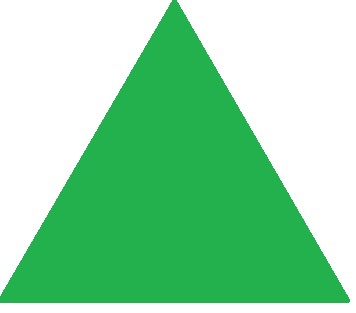Regular Triangle- Equilateral Triangle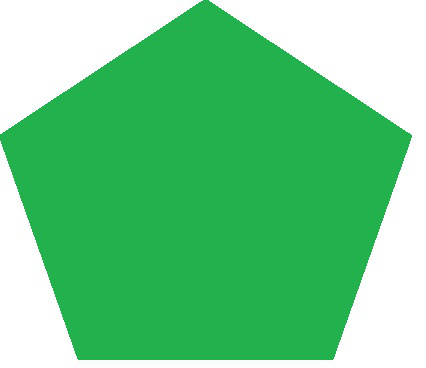Pentagon – Regular Pentagon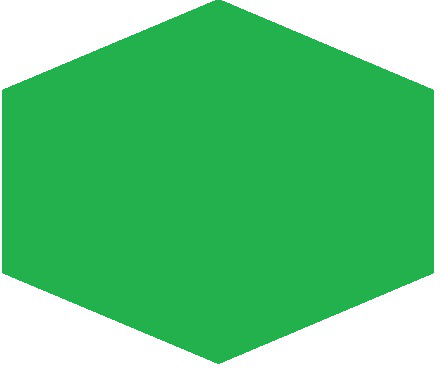Hexagon -Regular Hexagon

Question: What is the exterior and interior angle of a regular hexagon?

As we know regular hexagon is formed by joining 6 line segments so number of sides in hexagon is 6.

Exterior AngleInterior angle = 180° – exterior angle = 180° – 60° = 120°

A polygon having equal sides and equal angles is a regular polygon.

Area of regular polygon =(½) x Perimeter x Apothem

Example: Find the area of the regular polygon if the perimeter of the polygon is 20 cm and Apothem is 5 cm.

Solution:

Area of regular polygon =(½) x 20 cm x 5 cm = 50 cm2

### Irregular Polygon

Think about a polygon having different angles and different sides. You cannot find out the area of this polygon as a regular polygon. Such type of polygons are defined as irregular polygons.

Vertices– A point where two or more lines meet is called a vertex. The point of intersection of edges denotes the vertices.

Sides- Lines segment which is used to form a polygon, it’s denoted by n. Here in irregular polygon length of each side is not equal.

Exterior angle- The sum of the exterior angles of an irregular polygon also equals 360° but the angles are not equivalent. Because irregular polygons have interior angles with different measurements, because of that each exterior angle has a different measurement as well.

### Area of Irregular Polygon

To find the area of an irregular polygon you first separate the shape into regular. Then use the regular polygon area formulas to find the area of each of those polygons. Later add the area of all regular polygons to get the area of an irregular polygon.

To find the area of a triangle, we use the following formula:

Area of triangle=(1/2) x Base x Height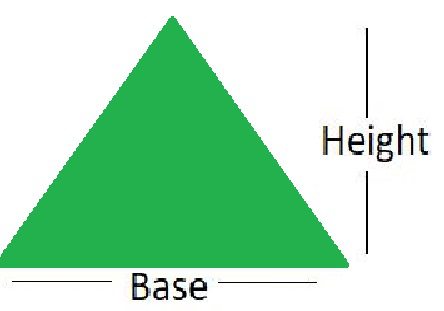To find the area of a quadrilateral, we can divide the quadrilateral into two triangles by constructing a diagonal and find the area of the triangles and then add them to find the area of the given quadrilateral.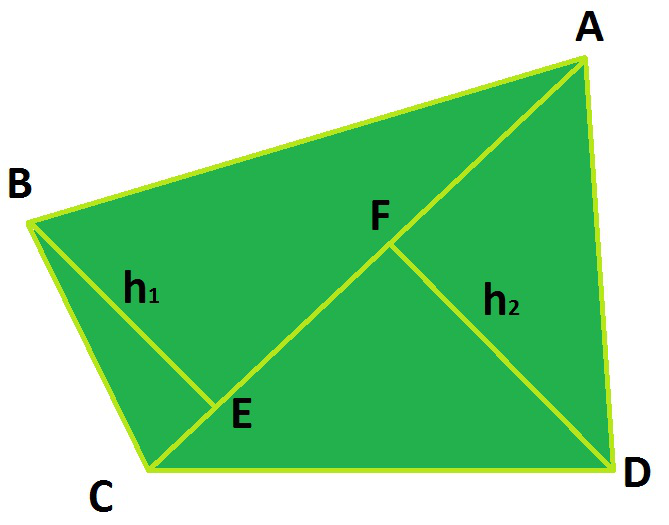Area of quadrilateral = (Area of Triangle)1 + (Area of Triangle)2

In the above figure, the diagonal AC will act as a common base of the two triangles ABC and ADC with heights h1 and h2 respectively.

Area of quadrilateral (ABCD) = Area of Triangle (ABC) + Area of Triangle (ACD)

Area of quadrilateral (ABCD) = (1/2) x AC x h1 + (1/2) x AC x h2

Area of quadrilateral (ABCD) = (1/2) x AC x (h1 + h2)

Similarly, to find the area of a Pentagon, Hexagon, Heptagon, and Octagon or for any other polygon, we will divide the polygon into different figures and then add the area of each figure to calculate the area of the polygon.

Here, we are taking an example of a pentagon ABCDE and we will find its area by dividing it into four parts.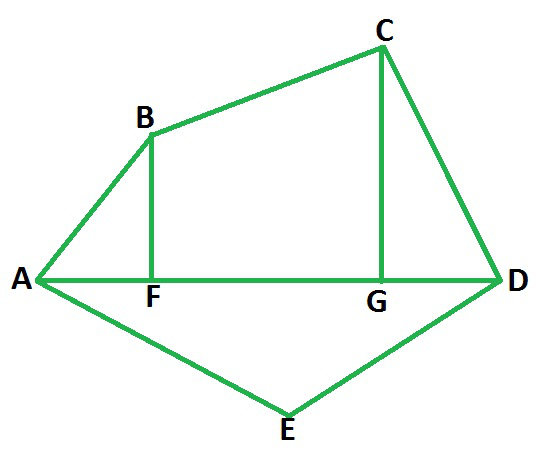By constructing one diagonal AD and two perpendiculars BF and CG on it, pentagon ABCDE is divided into four parts. So,

Area of Pentagon (ABCDE) = Area of right angled ∆ AFB + Area of trapezium (BFGC) + Area of right angled ∆ CGD + Area of ∆ AED

Similarly, we can find the area of any polygon by dividing the polygon into different parts.

### Sample Problems

Now, we will do some questions related to finding the area of polygon and this will help us clear the concept in a much better manner.

Question 1: Find the area of the given polygon.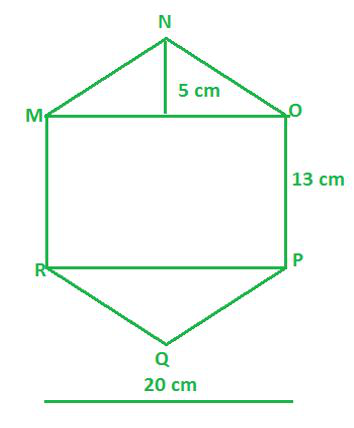Solution:

We are given the above figure which is a Hexagon and we are supposed to find out the area of the above figure MNOPQR.

Area of the figure MNOPQR = Area of triangle MNO + Area of triangle PQR + Area of rectangle MOPR

Area of the figure MNOPQR = (1/2) × MO × NK +(1/2) × PR × QL + PO × MO

Area of the figure MNOPQR = (1/2) × 20 × 5 + (1/2) x 20 × 5 + 13 × 20

Area of the figure MNOPQR = 10 × 5 + 10 × 5 + 260

Area of the figure MNOPQR = 50 cm2+ 50 cm2 + 260 cm2

Area of the figure MNOPQR = 360 cm2

Hence, the area of the given Hexagon MNOPQR is 360 cm2.

Question 2: Find the area of the given polygon.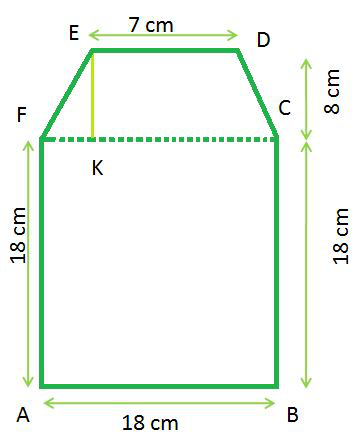Solution:

We are given the above figure which is a Hexagon and we are supposed to find out the area of the above figure ABCDEF.

Area of the figure ABCDEF = Area of Trapezium DEFC + Area of Square ABCF

Area of the figure ABCDEF = (1/2) × (ED+FC) × EK + (AF)2

Area of the figure ABCDEF = (1/2) × (7 + 18) × 8 + (18)2

Area of the figure ABCDEF = 4 × 25 + 18 × 18

Area of the figure ABCDEF = 100 cm2 + 324 cm2

Area of the figure ABCDEF = 424 cm2

Hence, the area of the given Hexagon ABCDEF is 424 cm2

Question 3: Find the area of the given polygon.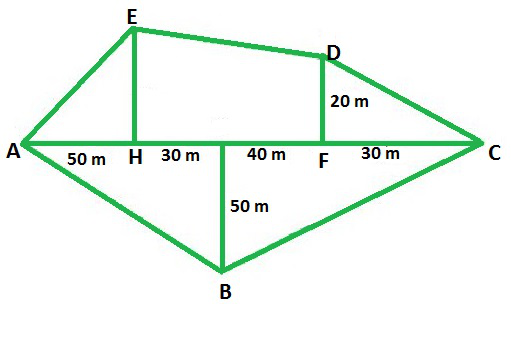Solution:

We are given the above figure which is a Pentagon and we are supposed to find out the area of the above figure ABCDE.

Area of the figure ABCDE = Area of triangle AHE + Area of Trapezium DEHF + Area of triangle DFC + Area of Triangle ABC

Area of the figure ABCDE = (1/2) × AH × HE + (1/2) × (EH+DF) × HF + (1/2)× FC × DF + (1/2) × AC × GB

Area of the figure ABCDE = (1/2) × 50 × 30 + (1/2) × (30+20) × 70 + (1/2) × 30 × 20 + (1/2) × 150 × 50

Area of the figure ABCDE = 25 × 30 + 50 × 35 + 15 × 20 + 150 × 25

Area of the figure ABCDE = 750 m2 + 1750 m2+ 300 m2+ 3750 m2

Area of the figure ABCDE = 6550 m2

Hence, the area of the given Pentagon ABCDE is 6550 m2.

Question 4: Find the area of the given polygon, where the length of the diagonal AC is 18 cm.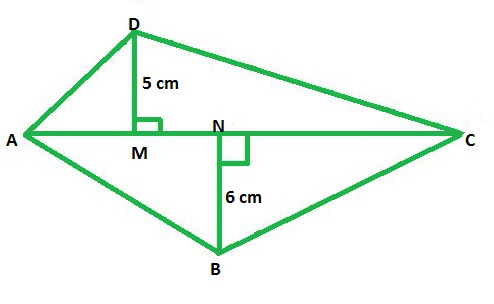Solution:

We are given the above figure which is a quadrilateral, and we are supposed to find out the area of the above figure ABCD.

Area of the quadrilateral ABCD = Area of triangle ABC + Area of triangle ADC

Area of the quadrilateral ABCD = (1/2) × AC × DM + (1/2) × AC × BN

Area of the quadrilateral ABCD = (1/2) × AC × (BN+DM)

Area of the quadrilateral ABCD = (1/2) × 18 × (6+5)

Area of the quadrilateral ABCD = 9 × 11 cm2

Area of the quadrilateral ABCD = 99 cm2

Hence, the area of the given quadrilateral is 99 cm2.

Question 5: Find the area of the given polygon.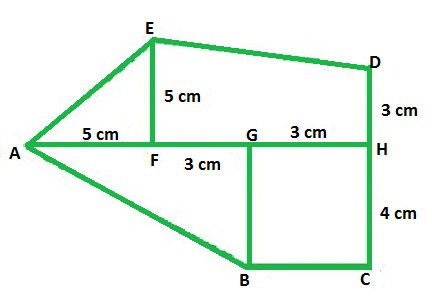Solution:

We are given the above figure which is a Pentagon and we are supposed to find out the area of the above figure ABCDE.

Area of the figure ABCDE = Area of triangle AGB + Area of Rectangle BCHG + Area of triangle AFE + Area of Trapezium DEFH

Area of the figure ABCDE = (1/2) × AG × BG + BG × GH + (1/2) × AF × FE + (1/2) × (DH+EF) × FH

Area of the figure ABCDE = (1/2) × 8 × 4 + 4 × 3 + (1/2) × 5 × 5 + (1/2) × (3+5) × 6

Area of the figure ABCDE = 4 × 4 + 4 × 3 + 2.5 × 5 + 3 × 8 cm2

Area of the figure ABCDE = 16 + 12 + 12.5 + 24 cm2

Area of the figure ABCDE = 64.5 cm2

Hence, the area of the given Pentagon ABCDE is 64.5 cm2.

Question 6: Find the area of the given polygon.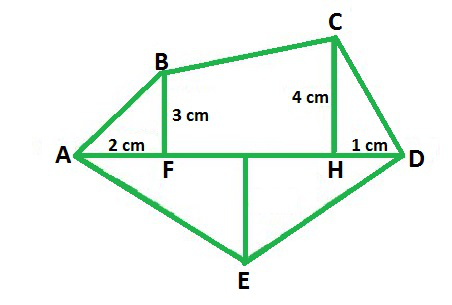Solution:

We are given the above figure which is a Pentagon, and we are supposed to find out the area of the above figure ABCDE.

Area of the figure ABCDE = Area of triangle ADE + Area of triangle CHD + Area of Trapezium BCHF + Area of triangle ABF

Area of the figure ABCDE = (1/2) *AD*GE + (1/2) *HD*CH + (1/2) *(CH+BF)*FH + (1/2) *AF*BF

Area of the figure ABCDE = (1/2) × 7 × 4 + (1/2) × 1 × 4 + (1/2) × (4+3) × 4 + (1/2) × 2 × 3 cm2

Area of the figure ABCDE = 2 × 7 + 2 + 7 × 2 + 1 × 3 cm2

Area of the figure ABCDE = 14 + 2 + 14 + 3 cm2

Area of the figure ABCDE = 33 cm2

Hence, the area of the given Pentagon ABCDE is 33 cm2.

My Personal Notes arrow_drop_up